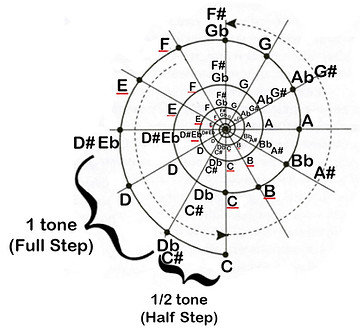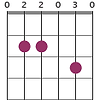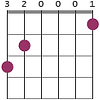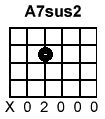Building chords:

Chords are 3 or more notes played at the same time, in order to build them you first need to know which major scale the chord is taken from.

Major scale structure:

The major scale is built from eight notes. The eighth note is actually the first note an octave higher.

The first note of the scale is called the ‘root’ it holds the name of the scale and you start and end the scale with it.

The distances between the notes determine which note are used for that specific scale. The distances are fixed as such:

Root +6 tones

X     1     X     1     X     1/2      X     1     X     1     X     1     X     1/2     X

(tone)     (tone)      (tone)        (tone)     (tone)      (tone)     (tone)

*On the guitar each fret is a semitone above the previous one.

So, if I want to build the scale of C major, it will look like this

C     1     D     1     E     1/2     F     1     G     1     A     1      B      1/2      C (+6) Root

When building a chord you MUST use the scale which bares the name of the chord, thus:

If I want to build the chord: C major, I would reference to the C major scale. If I want to build the chord Cm7 I would also go to the C major scale. But, If I want to build the chord F#m for instance, I would go to the F# major scale.

Chords formulas:

So, in order to build a chord you need to know which notes to choose from it’s scale.

For that we have chord “formulas”, those formulas indicate which notes you need to use.

In order to understand the formulas you have to know the numbers we assign to each note on the scale.

The numbers are basically arranged by the order the notes are arranged (excluding the eighth note) and we use Roman Numerals).

So in the scale of C major:

I     II    III    IV    V    VI    VII

C   D     E     F    G    A      B

So now you are ready to use the chord formulas:

Let’s say you want to build a major chord; A major chord is built from 3 notes: the first (I) the third (III) and the fifth (V).

Now let’s say we decided to build the C major chord.

The first step is to build its major scale (C major scale) which we have already done above.

The next step is to take out the three notes we need. In this case the I = C, the III = E and the V = G

So the chord C major is built from C E G (remember that the C is the root AND the bass note - this means you never change the lowest note that you play. If you do, you are changing the name of the chord as well).

Let’s try another one:

Let’s say I want to play Dm (minor);

First step - built the D major scale;

D  1  E   1  F#   ½   G   1   A   1    B   1   C#   ½   D

*The reason we have different notes now is because the distance between E & F is only a semitone and also between      B & C. (As shown in the images below).

Second step: identify which notes you need to take.

In this case: The formula is I III b and V, The b means that you take the original third note and lower it by half a tone, so in this case an F# would become an F.

*If for example the third would be an A than you would have lowered it to Ab.

So the Dm chord would be constructed from: I (D)   the III b (F)  & the V (A)

So now for some chord formulas:

X (Major) - I    III   V

Xm (minor) - I    IIIb   V

X7 (dominant 7) - I   III   V   VIIb

Xm7 (minor 7) - I   IIIb   V   VIIb

Xmaj7 - (major 7) - (sometimes seen like so: X7+ or X△7) - I   III   V    VII

Xm7+ - I   IIIb V  VII

Xsus2 - I   II   V - notice that this chord does not have a III

Xsus4 - I   IV  V - notice that this chord does not have a III

X6 - I   III   V   VI

Now, How do we take it to the guitar?

If you encounter a chord you do not know how to play on the guitar, the first step is to build it on paper.

So let’s say your new chord is Am7.

step 1: build it’s scale (in this case A major scale)

A   B   C#   D   E   F#   G#   A

Step 2: take out the notes that comprise Am7, using the correct formula (I  IIIb VIIb).

A  C  E  G (not a C# or a G# because both the three and the seven are flattened).

Step 3: Take the guitar and hold the most basic formation of that chord that you do know. So let’s say we take A.

Now, which notes is A built from? According to the formula of a major scale it’s; I (A) III (C#)  V (E). So now we need to make the changes we need to convert this A into an Am7.

There are two steps here:

1. Change every C# you are playing to a C (that would create the minor part of the chord)

2. add at least 1 G to the chord (that would add the 7’th note).

So A looks like this on the guitar:

Step 4:

Starting from the lowest string that you play (in this case the 5th, since you are not supposed to play the 6th).

Identify what notes you are playing:

5th string open is an A

4th string second fret is an E

3rd string second fret is another A

2nd string second fret is a C#

1st string open is another E

Step 5: making the changes:

So as we mentioned above, we cannot change the bass string (in this example it’s the open 5th string, the note A).

So where can we create the note G and where can we change the note C# into a C?

The 4th string is now an E, when it’s open it’s a D - we cannot use that. G is three semitones away from E that would be the 5th fret and would be very uncomfortable to play. So we leave it be.

The 3rd string is now an A when it’s open it’s a G - We already got the A on the open 5th string, so we are not losing any notes, perfect!

The 2nd string is now C#, if we move our finger down by one fret we create a C (our minor)

1st string is an E and does not require changes.

That’s it you're done! Rearrange your fingers to the most comfortable position and that would be your new chord.

Some tips:

In order to quickly identify the notes you need:

Major chord:

Between the I and the III there are always 2 tones, so If my I is E my III would be G.

Between the III and the V there are always 1 and a ½ tones. so If my III is an A my V would be C

Minor chord is built with the exact reversed distances.

1 & ½ tones III 2 tones

chords with a 7:

In order to find the 7th notes just go back 1 tone from your root in the scale. so if your I is C your VII will be Bb.

Chords with a maj7:

In order to find the 7th notes just go back ½ tone from your root in the scale. so if your I is C your VII will be B.

9th: are actually the 2nd note an octave higher

11th: are the 4th note an octave higher

13th: are the 6th note an octave higher

Sus2:

Change the major 3 by going 1 tone down - so if your III is F# your sus2 is an E

sus4:

Change the major 3 by going ½  tone up - so if your III is F# your sus4 is a G.

Now, let’s practice.

Build the following chords on your guitar:

*TIP:  X/X means you change the bass (root) note you play into what comes after the ' ' .

## Root + 6 TonesFmaj7Amaj7Csus2Gsus4Em7Dmaj7Cmaj7G7Csus4B7Am/CA7sus2C/BEsus4D7sus4